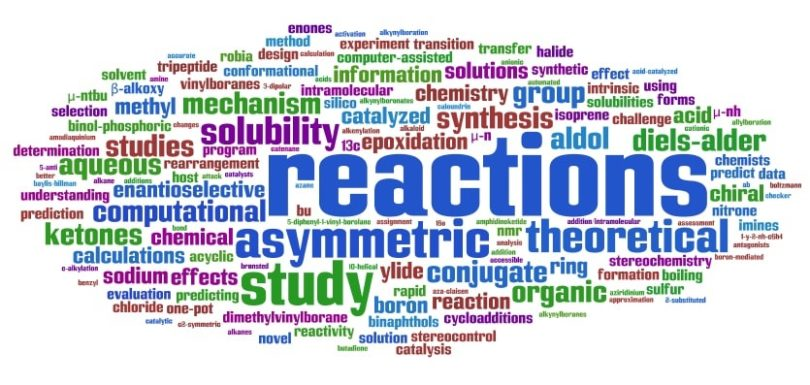# Atomic Number and Mass number practice QuestionsQues. The number of electrons in an atom of an element is equal to its
(a) Atomic weight
(b) Atomic number
(c) Equivalent weight
(d) Electron affinity

Ans. (b)

Ques. The nucleus of the element having atomic number 25 and atomic weight 55 will contain
(a) 25 protons and 30 neutrons
(b) 25 neutrons and 30 protons
(c) 55 protons
(d) 55 neutrons

Ans. (a)

Ques. If W is atomic weight and N is the atomic number of an element, then
(a) Number of e–1 = W – N
(b) Number of 0n1 = W – N
(c) Number of 1H1 = W – N
(d) Number of 0n1 = N

Ans. (b)

Ques. The total number of neutrons in dipositive zinc ions with mass number 70 is
(a) 34
(b) 40
(c) 36
(d) 38

Ans. (b)

Ques. The mass of an atom is constituted mainly by
(a) Neutron and neutrino
(b) Neutron and electron
(c) Neutron and proton
(d) Proton and electron

Ans. (c)

Ques. The atomic number of an element represents
(a) Number of neutrons in the nucleus
(b) Number of protons in the nucleus
(c) Atomic weight of element
(d) Valency of element

Ans. (b)

Ques. An atom has 26 electrons and its atomic weight is 56. The number of neutrons in the nucleus of the atom will be
(a) 26
(b) 30
(c) 36
(d) 56

Ans. (b)

Related: Logarithm questions

Ques. Number of electrons in the outermost orbit of the element of atomic number 15 is
(a) 1
(b) 3
(c) 5
(d) 7

Ans. (c)

Ques. The atomic weight of an element is double its atomic number. If there are four electrons in 2p orbital, the element is
(a) C
(b) N
(c) O
(d) Ca

Ans. (c)

Ques. An atom has the electronic configuration of 1s2, 2s2 2p6, 3s2 3p6 3d10, 4s2 4p5. Its atomic weight is 80. Its atomic number and the number of neutrons in its nucleus shall be
(a) 35 and 45
(b) 45 and 35
(c) 40 and 40
(d) 30 and 50

Ans. (a)

Ques. Compared with an atom of atomic weight 12 and atomic number 6, the atom of atomic weight 13 and atomic number 6
(a) Contains more neutrons
(b) Contains more electrons
(c) Contains more protons
(d) Is a different element

Ans. (a)

Ques. Ca has atomic no. 20 and atomic weight 40. Which of the following statements is not correct about Ca atom
(a) The number of electrons is same as the number of neutrons
(b) The number of nucleons is double of the number of electrons
(c) The number of protons is half of the number of neutrons
(d) The number of nucleons is double of the atomic number

Ans. (c)

Ques. The atomic number of an element having the valency shell electronic configuration 4s2 4p6 is
(a) 35
(b) 36
(c) 37
(d) 38

Ans. (b)

Ques. The present atomic weight scale is based on
(a) C12
(b) O16
(c) H1
(d) C13

Ans. (a)

Ques. If the atomic weight of an element is 23 times that of the lightest element and it has 11 protons, then it contains
(a) 11 protons, 23 neutrons, 11 electrons
(b) 11 protons, 11 neutrons, 11 electrons
(c) 11 protons, 12 neutrons, 11 electrons
(d) 11 protons, 11 neutrons, 23 electrons

Ans. (c)

Ques. The atomic number of an element is always equal to
(a) Atomic weight divided by 2
(b) Number of neutrons in the nucleus
(c) Weight of the nucleus
(d) Electrical charge of the nucleus

Ques. The mass number of an anion, X3–, is 14. If there are ten electrons in the anion, the number of neutrons in the nucleus of atom, X2 of the element will be
(a) 10
(b) 14
(c) 7
(d) 5

Ans. (c)

Ques. Number of unpaired electrons in inert gas is
(a) Zero
(b) 8
(c) 4
(d) 18

Ans. (a)

Ques. Nitrogen atom has an atomic number of 7 and oxygen has an atomic number 8. The total number of electrons in a nitrate ion will be
(a) 8
(b) 16
(c) 32
(d) 64

Ans. (c)

Ques. If molecular mass and atomic mass of sulphur are 256 and 32 respectively, its atomicity is
(a) 2
(b) 8
(c) 4
(d) 16

Ans. (b)

Ques. The atomic number of an element is 17. The number of orbitals containing electron pairs in its valence shell is
(a) Eight
(b) Six
(c) Three
(d) Two

Ans. (c)

Ques. The atomic number of an element is 35 and mass number is 81. The number of electrons in the outer most shell is
(a) 7
(b) 6
(c) 5
(d) 3

Ans. (a)

Ques. The number of neutron in tritium is
(a) 1
(b) 2
(c) 3
(d) 0

Ans. (b)

Ques. The atomic number of an element is 35. What is the total number of electrons present in all the p-orbitals of the ground state atom of that element
(a) 6
(b) 11
(c) 17
(d) 23

Ans. (c)

Related: d and f Block Elements MCQ

Ques. An element have atomic weight 40 and it’s electronic configuration is 1s2 2s2 2p6 3s2 3p6. Then its atomic number and number of neutrons will be
(a) 18 and 22
(b) 22 and 18
(c) 26 and 20
(d) 40 and 18

Ans. (a)

Ques. Which of the following is always a whole number
(a) Atomic weight